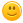+ Нов въпросandrey_bg 4 Точки

Lists Advanced - More Exercises/ 05. Kate's Way Out

Здравейте колеги,

имам проблем с това лабиринтче-въртя суча и не мога повече от 60/100 точки да изкарам, като ми гърми с runtime error на 3-ти и 4-ти опит, а при първото си решение 4-ти ми беше верен, така че предполагам че някои гранични случаи пропускам.

Ще бъда благодарен ако някой може да ми помогне!Условие на задачата:

4.Kate's Way Out

Kate is stuck into a maze, you have to help her to find her way out

On the first line you will be given how many rows there are in the maze. On the next n lines you will be given the maze itself. Here is a legend for the maze:

• "#" - means a wall; Kate cannot go through there
• " " - means empty space; Kate can go through there
• "k" - the initial position of Kate; start looking for a way out from there

There are two options: Kate either gets out or not. If Kate can get out print the following:
"Kate got out in {number_of_moves} moves". Otherwise print: "Kate cannot get out"

Examples

 Input Output 4 ###### ##  k# ## ### ## ### Kate got out in 5 moves 5 ###### ##  k# ## ### ###### ## ### Kate cannot get out

Моето решение:

rows=int(input())
maze=[list(input()) for i in range(rows)]
start=[(row,col) for row in range(rows) for col in range(len(maze[row])) if maze[row][col]=="k"]

exitPoint=[(row,col) for row in range(rows) for col in range(len(maze[row])) if maze[row][col]==" " and
(row==rows-1 or row==0 or col==0 or col==len(maze)-1)]
washere=[]; correctPath=[]

for row in range(rows):
washere.append([])
correctPath.append([])
for col in range(len(maze[row])):
washere[row].append(False)
correctPath[row].append(False)

def recursiveSolve(x, y):
if x == exitPoint and y== exitPoint: return True  #If you reached the end
elif maze[x][y] == "#" or washere[x][y]: return False   #If you are on a wall or already were here

# mark as visited
washere[x][y]=True

if x != 0: # Checks if not on top edge
if recursiveSolve(x - 1, y): # Recalls method one up
correctPath[x][y] = True # Sets that path value to True;
return True

if x != rows  - 1: # Checks if not on bottom edge
if recursiveSolve(x+1, y): # Recalls method one down
correctPath[x][y] = True
return True

if y != 0: # Checks if not on left edge
if recursiveSolve(x, y-1): # Recalls method one to the left
correctPath[x][y] = True
return True
if y != len(maze[x]) - 1: # Checks if not on right edge
if recursiveSolve(x, y+1): # Recalls method one to the right
correctPath[x][y] = True
return True
return False;

counter=1
res=recursiveSolve(start,start)
counter+=sum(sum(row) for row in correctPath)
if not res:
print("Kate cannot get out")
else:
print(f"Kate got out in {counter} moves")
Тагове:
0
22/02/2020 13:22:16vigyriousx 10 Точки

В exitpoint не проверяваш дали Кейт не седи там вече.

Пример:

##k##

## ##

#####

0
28/12/2020 16:03:27MomchilAntonov 2 Точки

Колеги, дайте едно читаво решение на тази задача. Направо ме съсипа :)

0matani 0 Точки

Колеги, мъча я таз' гад от два дни... положението е следното:

https://pastebin.com/675QXdQD

Но, искам да обърна внимание на ред 36, в който ред с проверки нарочно премахнах проверката х == 0, тоест, в този случай, проверка, която работи, ако Кейт е на първия ред на картата и може да излезе с един ход нагоре. Е, противно на всякаква логика с тази проверка - резултатът в Джъдж е 60/100, без нея 100/100.

Не знам, може би някъде нещо не е наред и съм омазал, но аз лично капитулирам и минавам нататък.  :)

0leonidg 0 Точки

Здравейте,

Началният ми подход беше като в първия пост, но се оказа адски трудно за дебъгване (поне за мен). Затова седнах и го разписах като първолак:

https://pastebin.com/TAEAfFNN

0raogma 0 Точки

И аз я реших, като махнах проверката за редовете == 0. Според мен имат грешка с тестовете.

Аз ползвах рекурсия, тъй като става малко по - прегледно:

https://pastebin.com/WSV8Bxrt

0